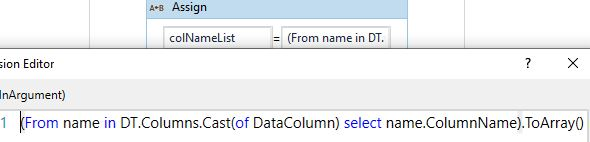# How to loop through N column in a row

In a macro enabled excel sheet I need to get the data from row2 & row3 for N columns. every time i need to get the data from C3,C4 next D3,D4 next E3,E4 …till n3,n4 that loops through 2 rows and N columns in a excel sheet can you please help me to get the loop concept for this

say datatable variable name is :dt
use a for each activity and in argument give: dt.columns
dt.columns will give you a list of string of column names of the datatable
now you can loop though each column for each row using row(columnIterator)

Can u please explain me a bit am not getting it

Hello @KKsekar ,

You can write as below to get the column names of the Datatable.So the concept will be, you need to use 2 loops.One for column and one for row
outer loop will be column and inner loop you have to use row.

Use for each activity and loop through the colNameList.
{
use for each activity for rowlist. ( {3,4})
{Use read cell activity and pass the value dynamically (Suppose if its C3 , C is the variable value in the Outer loop and 3 is the variable value in the inner loop). So you can append both to get the Cell Number.
}}

Loop will continue till the last value in the colNamelist.

I have used build datatable activity to loop through columns am getting the column values one by one but not able to loop through (3,4)th position for each column

Ok…if that is the case then i hope you are using Foreach row in datatable activity and getting the Column value. So that will be the first loop. Inside that as i mentioned create an another for each loop for the array {3,4}.

As you mentioned you need to get the value from C3,C4,D3,D4,E3,E4 etcccc).

So First loop( for each row in datatable will give you the C,D,E,F etc) and second loop( for each will give you 3,4).

Suppose in the foreach row in datatable the variable is currentRow and in the inner loop for each variable is row.

In the ReadCell activity you will have to give currentRow.ToString+row.ToString

Thanks Rahul…Will try it now

Thanks @Rahul_Unnikrishnan It worked…

1 Like

This topic was automatically closed 3 days after the last reply. New replies are no longer allowed.